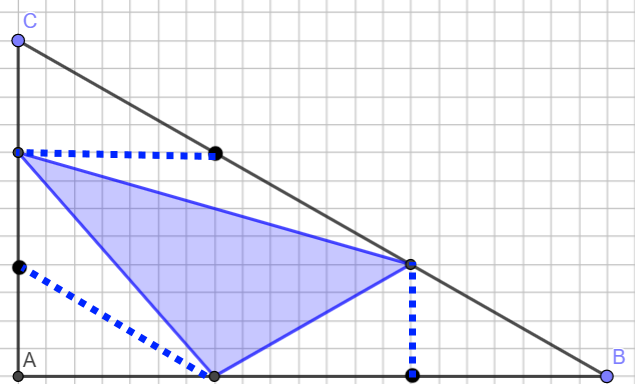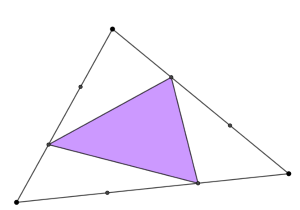You may also likeFitting In

The largest square which fits into a circle is ABCD and EFGH is a square with G and H on the line CD and E and F on the circumference of the circle. Show that AB = 5EF. Similarly the largest equilateral triangle which fits into a circle is LMN and PQR is an equilateral triangle with P and Q on the line LM and R on the circumference of the circle. Show that LM = 3PQLook Before You Leap

Can you spot a cunning way to work out the missing length?Triangle Midpoints

You are only given the three midpoints of the sides of a triangle. How can you construct the original triangle?

Triangle in a Triangle

Why do this problem?

In this problem, constructing some lines on a triangle divides the area in a surprising way. We hope that by trying some examples and calculating the areas, students will be intrigued by the result they notice, and curious to prove whether it will always work. Along the way, they will have plenty of opportunity to work with similar triangles, ratios and enlargements.

Possible approach

Begin by showing students a right-angled triangle such as the one below, with points marked $\frac13$ of the way along each side and joined to make a new triangle.

Invite them to draw some triangles, perhaps on squared paper, and calculate the areas. Once they have created some examples, bring the class together and share results. Perhaps students could add their results to a table on the board:

 Area of right-angled triangle Area of triangle inside

"What do you notice?" "The right-angled triangle's area is always three times the area of the inside triangle"

To prove the result, students might find it useful to add some extra lines to their diagram:As the triangles are right-angled, students could use similar triangles to explain why the small triangles at A, B and C are each $\frac19$ of the area of the whole triangle. Students can then explore the triangles with the same length base that share an apex; this gives us another three triangles with area $\frac19$. Students could be encouraged to write up this argument as a proof.

Alternatively, students who are familiar with vectors may like to express the lengths in the diagram in terms of the vectors $\overrightarrow{AB}$ and $\overrightarrow{AC}$, and then calculate the relevant areas.

Key questions

Which areas are easiest to calculate?
Can we use these areas to work out the shaded area?
Are there any extra points or lines we could add?

Possible extension

The problem uses a right-angled triangle for ease of area calculation, but the result is true for any triangle. For some students, it might be appropriate to skip straight to thinking about this general case:Students could then move on to Areas and Ratios, and Another Triangle in a Triangle

Possible support

Students could investigate Two Ladders to explore similar triangles.